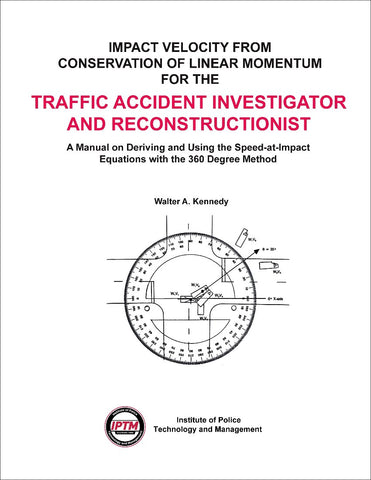# Impact Velocity from Conservation of Linear Momentum for the Traffic Accident Investigator and Reconstructionist

• \$ 25.00

Impact Velocity from Conservation of Linear Momentum
for the Traffic Accident Investigator and Reconstructionist

by W.A. Kennedy

This manual brings you the mathematical solutions to two-vehicle collisions that require the application of momentum. The author reduces the complex topic of linear momentum to a series of simple steps that use the 360-degree method of angle measurement and illustrates every step with easy-to-understand diagrams.

With this manual, you can learn how the speed-at-impact formulas are derived, what information is required for their use and how they can be applied to a variety of situations to solve for the pre-collision speed of each crash vehicle. The author, W. A. Kennedy, also discusses vehicle dynamics in collisions and lays the groundwork for the advanced study of momentum.

Six practical momentum problems enable you to test your understanding of the topic after you have read and studied the manual.

The book includes the following chapters:

• Conservation of Linear Momentum
• Newton’s Laws of Motion
• Data Required to Solve for Impact Velocity
• Cartesian Coordinate System
• Vectors
• Negative Vectors
• Determining Momentum
• Use of Subscripts to Identify Vehicles
• Change of Momentum Equation
• Derivation of Linear Momentum Equation
• Measuring Approach and Departure Angles
• Use of Trigonometric Functions
• Derivation of the Speed-at-Impact Equations from the Linear Momentum Equation
• Using the Speed-at-Impact Equations
• Practice Problems
• Use of Equipment

Walter A. “Butch” Kennedy served 17 years with the Jacksonville (Florida) Sheriff’s Department and was awarded the Medal of Merit for Bravery in 1983. While there, he was assigned as Academy instructor in crash investigation and reconstruction and tactical police driving. Mr. Kennedy has been a member of the faculty at IPTM teaching crash investigation and reconstruction courses throughout the country.

Specifications: 66 pages; 8½”x11”; Coil bound; Publisher: IPTM (June 1993)